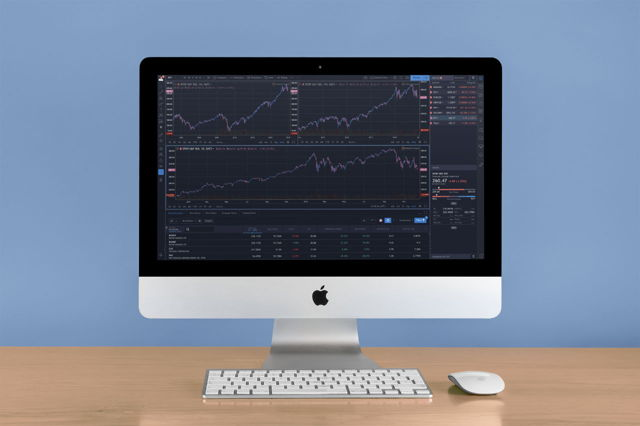# How to Calculate the Exponential Moving Average

To calculate the Exponential Moving Average, you need to obtain complete historical price data of the security you’re analyzing. Here you will find the formula to calculate the EMA.

•Matthias Hagemann

• Updated October 15th 2020

The formula for the Exponential Moving Average (EMA) is a cumulative calculation which includes all historical price data. Every EMA value of a period takes into consideration the EMA value of its preceding period.

Past values in the calculation have a diminishing weight to the EMA while more recent values have a greater weight.

The exact calculation with the EMA formula is as follows:

Where:

• n is the number of periods (e.g. 200 for the 200 EMA)
• EMA[-1] is the EMA of the previous period
• p is the price in the current period

## Exponential moving average formula

The smoothing constant K applies appropriate weight to the most recent price. It uses the number of periods n specified in the EMA. A 20 EMA applies a 9.52% weighting to the most recent price (2 / (20 + 1) = .0952). It is therefore also called a 9.52% EMA.

A 200 EMA applies a 0.995% weighting to the most recent price. Notice that the weighting for the shorter time period is more than the weighting for the longer time period. The weighting drops by half every time the moving average period doubles.

You can use variations of the EMA by using the open, high, low or median price instead of using the closing price by default.

## Same calculation for every time frame

The formula to calculate the 200 EMA is the same across all time frames, be it the daily, weekly, or even monthly time frame.

You can trade stocks using multiple time frames and greatly improve your profitability. The longer the time frame, the more reliable the stocks will react to its 200 EMA.Matthias Hagemann
Matthias’s career spans over a series of financial institutions. Trend following became a focus topic when he wrote his senior thesis on “The Psychology of Financial Markets”. He is portfolio manager at Hagemann Capital, a high-conviction investment fund.

Knowledge is power and helps us make difficult decisions intelligently. Here you will gain knowledge that will help you understand individual topics of trend following better.## Join the Live Chat

In the live chat, I will help you configure your charts so you have a really cool heads-up display. You’ll also receive 3 valuable lessons for a winning mindset. It’s all free!

## More Trend Following Posts### Read Price Action to Master Trend Following

Price action is the trading activity in a pricing process. It is used in trend following to understand how trends evolve and how they can be traded for profit.